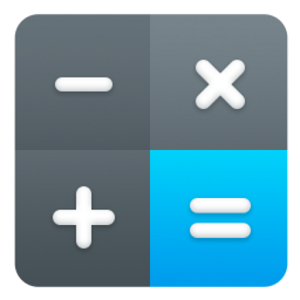Free
Overview System Requirements Related

## Description

Algebra Calculator is a free app equipped with powerful tools to solve basic algebraic equations as well as perform many mathematic functions, and it does it in style with the new Fluent Design UI. With Algebra Calculator, you can: - Solve algebraic equations given X - Get the prime factorization of any number - Solve quadratic equations - Expand factored quadratic equations - Solve and switch logarithms/exponential equations - Reduce radicals into their simplest form - Solve function compositions - Find the rule of arithmetic and geometric sequences - Solve recursive equations - Solve sigma equations - Search through a database of algebra, geometry, trigonometry, and physics formulas

Noku Development

Noku Development

07/06/2021

27.77 MB

#### Age rating

For ages 3 and over

#### This app can

Access all your files, peripheral devices, apps, programs and registry

#### Installation

Get this app while signed in to your Microsoft account and install on up to ten Windows 10 devices.

#### Language supported

English (United States)